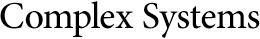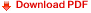## Cellular Automaton‐Based Emulation of the Mersenne TwisterKamalika Bhattacharjee${\text{}}^{*}$
Nitin More
Shobhit Kumar Singh
Nikhil Verma

Department of Computer Science and Engineering
National Institute of Technology
${\text{}}^{*}$corresponding author, kamalika.it@gmail.com
The Mersenne Twister (MT) (MT19937), developed 30 years ago, is the de facto pseudorandom number generator (PRNG) used in many computer programs. This paper proposes a candidate that offers a randomness quality that is better than MT19937 and its sisters SFMT19937 and TinyMT. A special three-neighborhood, two-state cellular automaton (CA), called CA $\left({150}^{\prime }\right)$ is the underlying model of this PRNG. The same working style of MT19937 is used, while avoiding the problems of the MT, like a large state space and the zero-access initial state problem. Nonlinearity is added in the base simple linear CA such that the properties of the base CA are not violated. Finally, a PRNG is developed using this CA that beats MT19937 as well as its advanced versions over the standard empirical platforms Dieharder, TestU01 and NIST.
Keywords: Mersenne Twister (MT); Sophie Germain prime; pseudorandom number generator (PRNG); maximal-length cellular automata; CA $\left({150}^{\prime }\right)$; nonlinearity